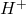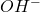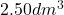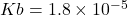pH is the measurement of hydronium ion concentration in the solution and it determines the acidity or basicity of the solution.When aqueous solutions of acid and base are mixed, the pH of the resultant solution depends on the concentration ofion left in the solution. Here are few steps to calculate pH of the solution after the completion of the reaction:

Step 1. First of all we need to know the volume and concentration of each component undergo mixing.

Step 2. Using the balanced chemical equation, number of moles of each component is calculated before mixing.

Step 3. Using the mole ratio, the number of moles of the excess component left in the solution is determined.

Step 4. The number of moles of the excess component is divided by the total volume of the solution to determine the new concentration of solution after mixing.

Step 5. If the excess component is a weak acid or weak base, then using the weak dissociation constant of acid or base, the concentration oforis determined.

Step 6. Using the concentration of, pH is calculated.

Let us understand the concept of calculating pH more clearly while solving a problem through the video.

This video covers the solution of a numerical problem: At, aof a 7.50 % HCl solution () are added of an NH3 solution at pH 10.85. Calculate the pH of the resulting solution.